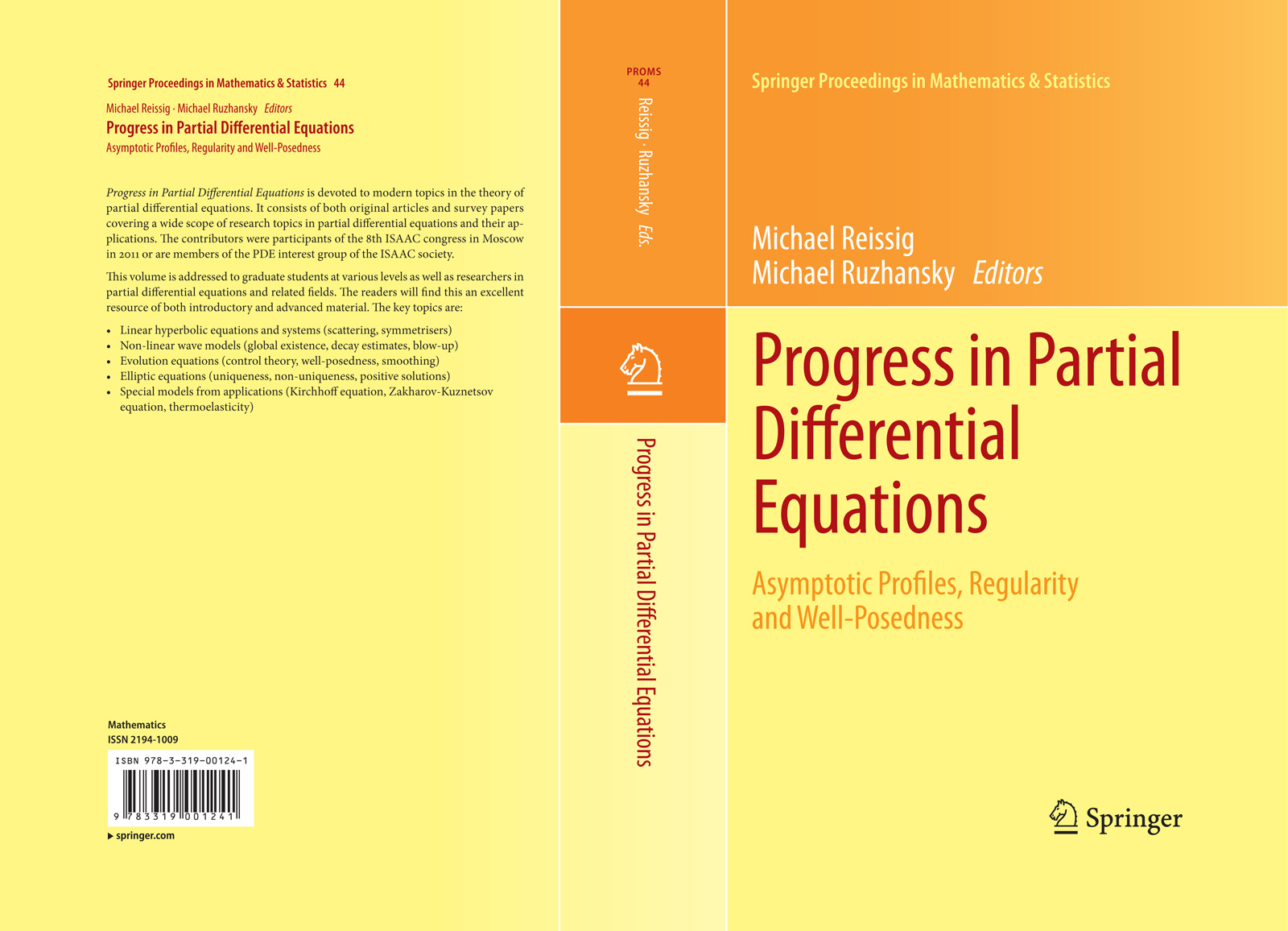• A
• A
• A
• ABC
• ABC
• ABC
• А
• А
• А
• А
• А
Regular version of the site

## On an Optimal Control Problem for the Wave Equation in One Space Dimension Controlled by Third Type Boundary Data

P. 223-238.

In the present paper we study the boundary control by the third boundary condition on the left end of a string, the right end being fixed. An optimality criterion based on the minimization of an integral of a linear combination of the control itself and its antiderivative raised to an arbitrary power p≥1 is established. A method is developed permitting one to find a control satisfying this optimality criterion and write it out in the explicit form. The optimal control for p>1 is proved. Thereby proposed optimality criterion uniquely determines the optimal solution of boundary control problem under consideration.

### In bookEdited by: M. Reissig. Vol. 44. Springer, 2013.